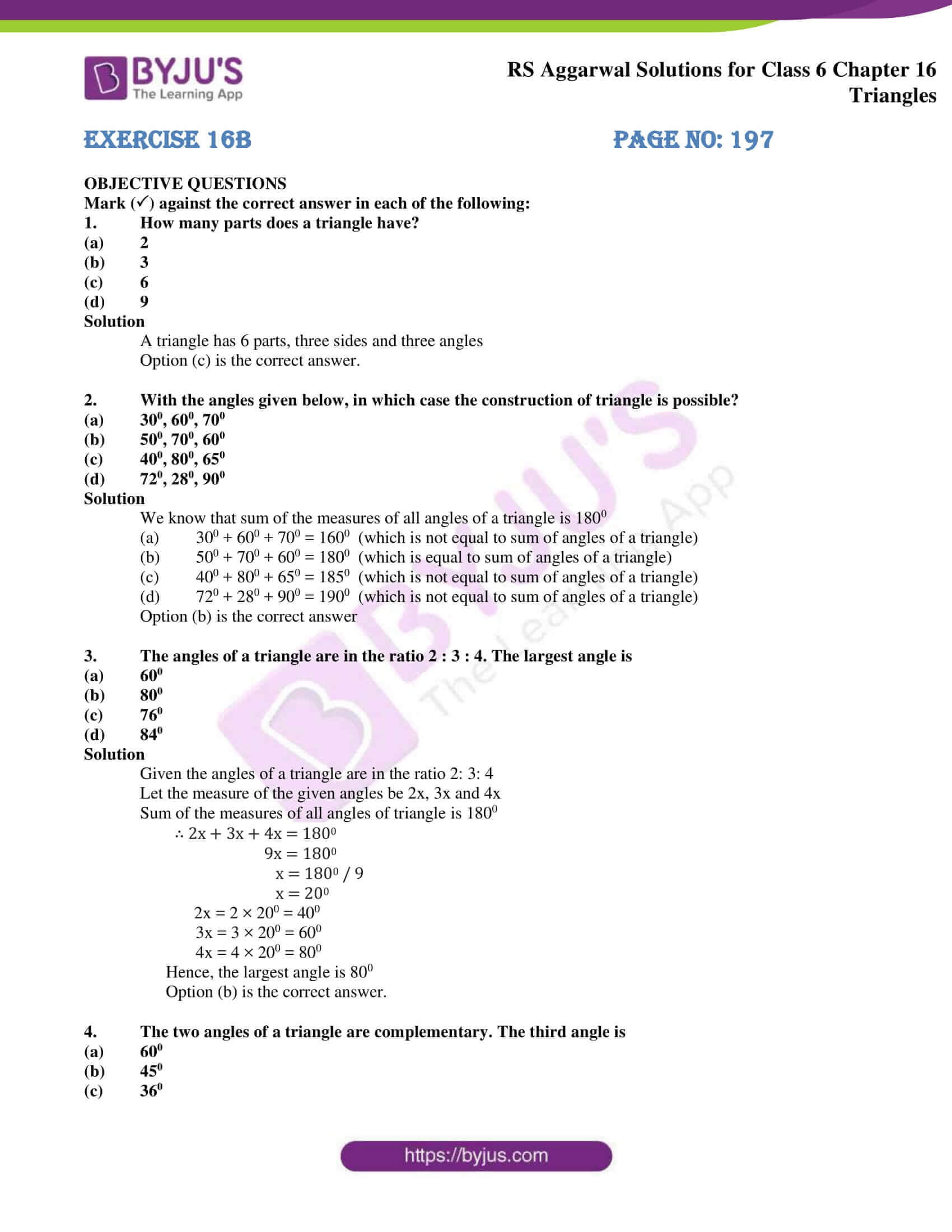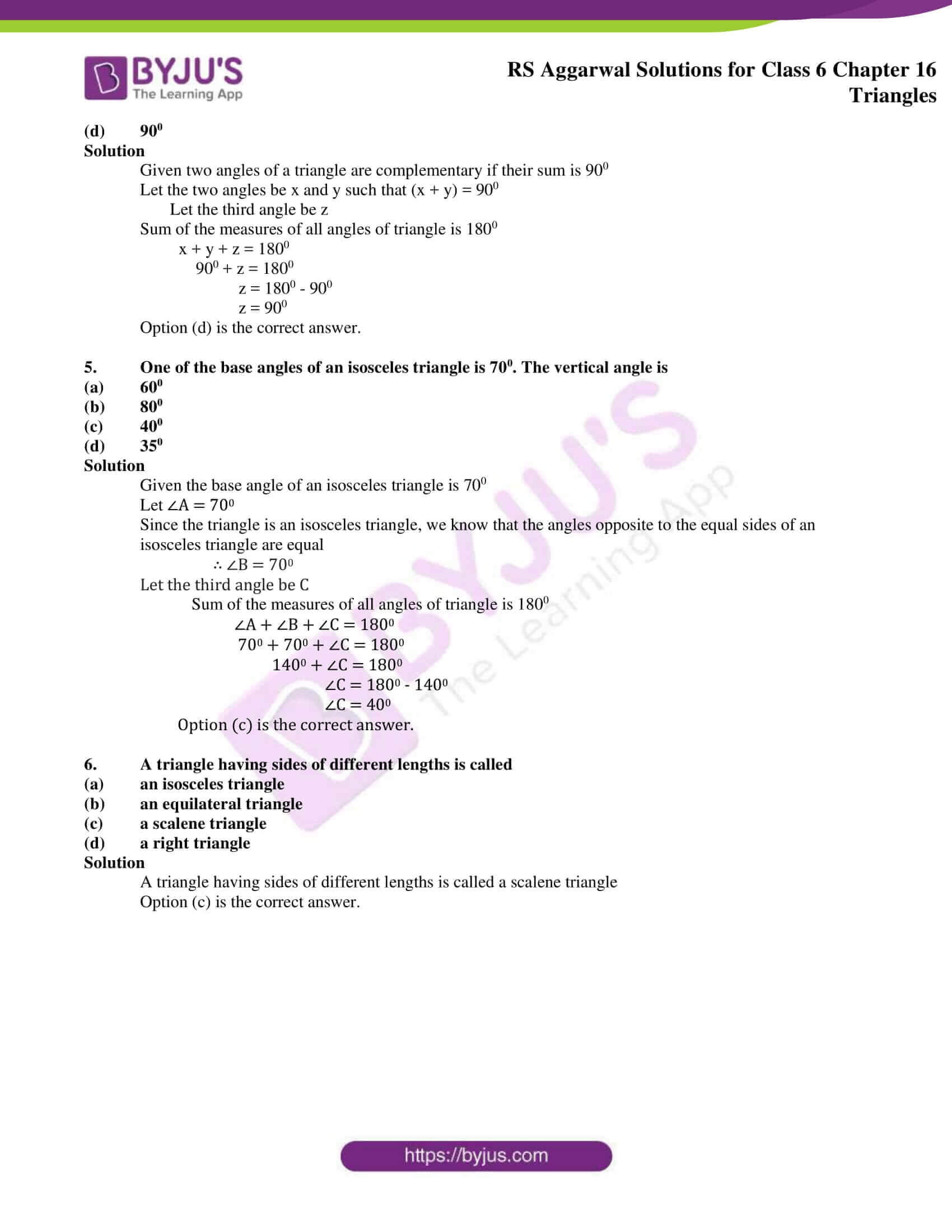# RS Aggarwal Solutions for Class 6 Maths Chapter 16 Triangles Exercise 16B

We at BYJU’S design the exercise solutions of RS Aggarwal textbook in such a way that it matches the understanding ability of students. The answers are prepared with simple shortcut methods which are used in solving tricky problems efficiently. The students can improve their speed in solving problems more accurately as per CBSE syllabus. RS Aggarwal Solutions for Class 6 Maths, Chapter 16 Triangles Exercise 16B PDF can be used by the students as a reference guide to ace the exam.

## Download PDF of RS Aggarwal Solutions for Class 6 Maths Chapter 16 Triangles Exercise 16B### Access answers to Maths RS Aggarwal Solutions for Class 6 Chapter 16 Triangles Exercise 16B

OBJECTIVE QUESTIONS

Mark () against the correct answer in each of the following:

1. How many parts does a triangle have?

(a) 2

(b) 3

(c) 6

(d) 9

Solution

A triangle has 6 parts, three sides and three angles

Option (c) is the correct answer.

2. With the angles given below, in which case the construction of triangle is possible?

(a) 300, 600, 700

(b) 500, 700, 600

(c) 400, 800, 650

(d) 720, 280, 900

Solution

We know that sum of the measures of all angles of a triangle is 1800

(a) 300 + 600 + 700 = 1600 (which is not equal to sum of angles of a triangle)

(b) 500 + 700 + 600 = 1800 (which is equal to sum of angles of a triangle)

(c) 400 + 800 + 650 = 1850 (which is not equal to sum of angles of a triangle)

(d) 720 + 280 + 900 = 1900 (which is not equal to sum of angles of a triangle)

Option (b) is the correct answer

3. The angles of a triangle are in the ratio 2 : 3 : 4. The largest angle is

(a) 600

(b) 800

(c) 760

(d) 840

Solution

Given the angles of a triangle are in the ratio 2: 3: 4

Let the measure of the given angles be 2x, 3x and 4x

Sum of the measures of all angles of triangle is 1800

∴ 2x + 3x + 4x = 1800

9x = 1800

x = 1800 / 9

x = 200

2x = 2 × 200 = 400

3x = 3 × 200 = 600

4x = 4 × 200 = 800

Hence, the largest angle is 800

Option (b) is the correct answer.

4. The two angles of a triangle are complementary. The third angle is

(a) 600

(b) 450

(c) 360

(d) 900

Solution

Given two angles of a triangle are complementary if their sum is 900

Let the two angles be x and y such that (x + y) = 900

Let the third angle be z

Sum of the measures of all angles of triangle is 1800

x + y + z = 1800

900 + z = 1800

z = 1800 – 900

z = 900

Option (d) is the correct answer.

5. One of the base angles of an isosceles triangle is 700. The vertical angle is

(a) 600

(b) 800

(c) 400

(d) 350

Solution

Given the base angle of an isosceles triangle is 700

Let ∠A = 700

Since the triangle is an isosceles triangle, we know that the angles opposite to the equal sides of an isosceles triangle are equal

∴ ∠B = 700

Let the third angle be C

Sum of the measures of all angles of triangle is 1800

∠A + ∠B + ∠C = 1800

700 + 700 + ∠C = 1800

1400 + ∠C = 1800

∠C = 1800 – 1400

∠C = 400

Option (c) is the correct answer.

6. A triangle having sides of different lengths is called

(a) an isosceles triangle

(b) an equilateral triangle

(c) a scalene triangle

(d) a right triangle

Solution

A triangle having sides of different lengths is called a scalene triangle

Option (c) is the correct answer.

### Access other exercises of RS Aggarwal Solutions for Class 6 Maths Chapter 16 Triangles

Exercise 16A Solutions